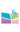﻿2020-08-24,朱佐农 教授,From integrability of spatial discrete Hirota equation to integrability of Hirota equation-中国矿业大学（北京）理学院网站2020-08-24,朱佐农 教授,From integrability of spatial discrete Hirota equation to integrability of Hirota equation

:  朱佐农 教授

摘     要：

Hirota equation and integrable spatial discrete Hirota equation are two very important integrable equations. In this talk, we will address the topic that from integrability of discrete Hirota equation to integrability of Hirota equation. This means that we will show how to get the integrability of the Hirota equation from the integrability of spatial discrete Hirota equation.This is a joint work with A. Pickering and H.Q. Zhao.

间： 2020824 4:00---5:30

式： 腾讯会议系统 ID824 899 920

密码：0824